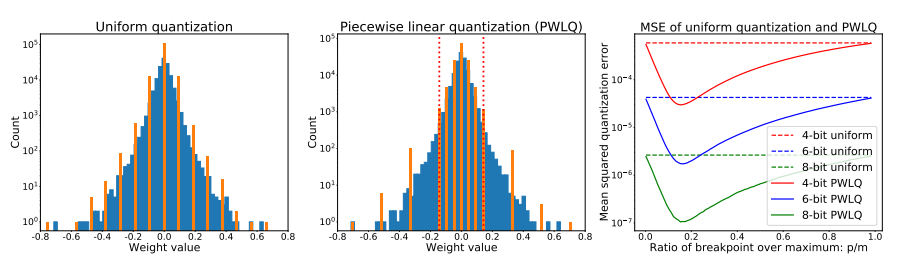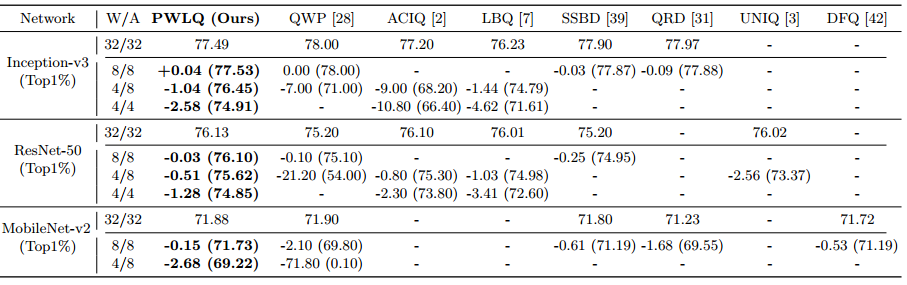# 浅谈深度学习模型量化

【GiantPandaCV】本次简要的总结了模型量化研究的一些问题，介绍了量化存在的量化误差与其总体上解决量化误差的一些方法。主要讨论了5种非线性量化的方法。

3、原精度即浮float-32，量化到什么样的数据类型，这里就有float和int；到底要选择量化后的是多少个bit，这里就有1-bit(二值网络)、2-bit(三值网络)、3-bit、4-bit、5-bit、6-bit、7-bit、8-bit，这几种量化后的数值类型是整型。

4、是固定所有网络都是相同的bit-width，还是不同的，这里就有混合精度量化(Mixed precision)

5、是从一个已经训练好的模型再进行量化，还是有fine tune的过程或者直接是从头开始训练一个量化的模型，这里就有Post-training quantization(后量化，即将已经训练完的模型参数进行量化)、quantization-aware training(量化感知训练，即在从头开始训练中加入量化)和quantization-aware fine tune(在fine tune训练中加入量化)。

1、从float-32到Int数据类型，其中有一个round的操作，这里肯定会有误差； 2、激活函数的截断； 3、溢出时候的处理也有可能带来误差。

1、weight和activation的数据分布呈现出一个类拉普拉斯分布或者类高斯分布，数据分布是一个钟型分布，大部分数据集中在中间，两头的数据比较少。如果采用均与量化(uniform quantization)，由于是等间隔的，那么中间密集分布的数据的分辨率低。举个例子，假如总共10个值，fp32_x={10，-10，0.11，0.21，0.15，0.05，-0.14，-0.22，-0.08，-0.35}，采用对称均匀分布量化，（10-（-10））/（255）= 20/255，Int8_x={127，-127，1，3，2，1，-2，-3， -1，-5}，可以看到，0.05和0.11被量化同一个int-8的数值1，而且量化后的数值大部分集中在了[-5,3]，而其余的数值没有用到。如何解决这个问题？很简单，给密集的区域用比较多的Int数值表示(增大分辨率)，稀疏的区域用比较少的Int数值表示；这就是一个不等间距间隔，称为非均与量化(non-uniform quantization)或非线性量化。

2、另外一个问题就是每一层的数值范围不一定都相同，activation在不同层的数值范围会不一样，这就会产生另外一个问题，动态值域问题，dynamic range。这个dynamic range如何影响量化效果呢？比如8-bit的均与分布量化，值域[-2, 2]的单位间隔是(2-(-2))/255 = 4/ 255，值域[-6, 6]的单位间隔是(6-(-6))/255 = 12/ 255，明显前面的分辨率更高。还是由于数据分布钟型分布，即可abs(数值)大的占据的比例很小，因此，这里可以采用截断的方式提高来量化的分辨率。

3、round会肯定会带来误差，怎么处理呢？Stochastic rounding，因为其期望是x，可以减少round的误差.

Round $(x)=\left\{\begin{array}{ll}\lfloor x\rfloor & \text { with probability } 1-(x-\lfloor x\rfloor) \\ \lfloor x\rfloor+1 & \text { with probability } x-\lfloor x\rfloor\end{array}\right.$

$E(x)= (1-(x-\lfloor x\rfloor)) * \lfloor x\rfloor + (x-\lfloor x\rfloor)*(\lfloor x\rfloor+1)$
$= \lfloor x\rfloor - (x-\lfloor x\rfloor) * \lfloor x\rfloor + (x-\lfloor x\rfloor)*\lfloor x\rfloor + (x-\lfloor x\rfloor)$
$= \lfloor x\rfloor + x-\lfloor x\rfloor = x$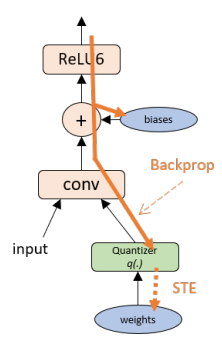2、 Powers-of-two quantization，这个方法我还没找到原论文
3、《Quantization Networks》CVPR 2019
4、《Additive powers-of-two quantization: an efficient non-uniform discretization for neural networks》 ICLR 2020
5、《Differentiable Soft Quantization: Bridging Full-Precision and Low-Bit Neural Networks》 CVPR 2019
6、《Post-Training Piecewise Linear Quantization for Deep Neural Networks》ECCV 2020

$s(a, b, n)$ 就是scale，$\left\lfloor\frac{\operatorname{clamp}(r ; a, b)-a}{s(a, b, n)}\right]$是量化，$\left\lfloor\frac{\operatorname{clamp}(r ; a, b)-a}{s(a, b, n)}\right] s(a, b, n)+a$是反量化，

\begin{aligned} \operatorname{clamp}(r ; a, b) &:=\min (\max (x, a), b) \\ s(a, b, n) &:=\frac{b-a}{n-1} \\ q(r ; a, b, n) &:=\left\lfloor\frac{\operatorname{clamp}(r ; a, b)-a}{s(a, b, n)}\right] s(a, b, n)+a \end{aligned}

$\mathcal{Q}^{u}(\alpha, b)=\alpha \times\left\{0, \frac{\pm 1}{2^{b-1}-1}, \frac{\pm 2}{2^{b-1}-1}, \frac{\pm 3}{2^{b-1}-1}, \ldots,\pm 1\right\}$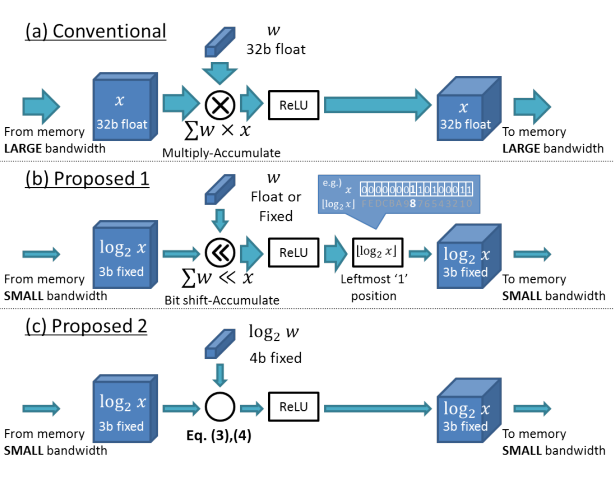\begin{aligned} w^{T} x & \simeq \sum_{i=1}^{n} w_{i} \times 2^{\tilde{x}_{i}} \\ &=\sum_{i=1}^{n} \operatorname{Bitshift}\left(w_{i}, \tilde{x}_{i}\right) \end{aligned}

\begin{aligned} w^{T} x & \simeq \sum_{i=1}^{n} 2^{\text {Quantize }\left(\log _{2}\left(w_{i}\right)\right)+\text { Quantize }\left(\log _{2}\left(x_{i}\right)\right)} \\ &=\sum_{i=1}^{n} \operatorname{Bitshift}\left(1, \tilde{w}_{i}+\tilde{x}_{i}\right) \end{aligned}

$\tilde{x}=\operatorname{Clip}\left(\operatorname{Round}\left(\log _{2}(|x|)\right), \mathrm{FSR}-2^{\text {bitwidth }}, \mathrm{FSR}\right)$

$\operatorname{Clip}(x, \min , \max )=\left\{\begin{array}{ll}0 & x \leq \min \\ \max -1 & x \geq \max \\ x & \text { otherwise }\end{array}\right.$

$\begin{array}{r} \text { LinearQuant }(x, \text { bitwidth, FSR }) \\ =\operatorname{Clip}\left(\text { Round }\left(\frac{x}{\text { step }}\right) \times \text { step }, 0,2^{\mathrm{FSR}}\right) \end{array}$

$Q_{P oT }(x)=\left\{\begin{array}{ll}\operatorname{sign}(x) & |x| \geq 1 \\ \operatorname{sign}(x) *2^{\left\lfloor\log _{2}|x|\right\rfloor} & 2^{-b+1} \leq|x|<1 \\ 0 & |x|<2^{-b+1}\end{array}\right.$

$\mathcal{Q}^{p}(\alpha, b)=\alpha \times\left\{0, \pm 2^{-2^{b-1}+1}, \pm 2^{-2^{j-1}+2}, \ldots, \pm 2^{-1},\pm 1\right\}$

$2^{x} r=\left\{\begin{array}{ll}r & \text { if } x=0 \\ r<0 \\ r>>x & \text { if } x<0\end{array}\right.$

$\gamma$表示是缩放系数，以保证$\mathcal{Q}^{a}$中的最大水平为$\alpha$
$k$是基位宽度(base bit-width)，是每个加法项的位宽；
$n$是加法项的数量。

2、PoT解决了一个问题，就是在值比较小的区域阶梯变多了，也就是分辨率变高了，但是也存在Powers-of-two quantization中提到的问题；
3、APoT就是为了解决PoT存在的问题，也就是当bit数增加的时候，两个最大值的之间的分辨率没有提高的问题。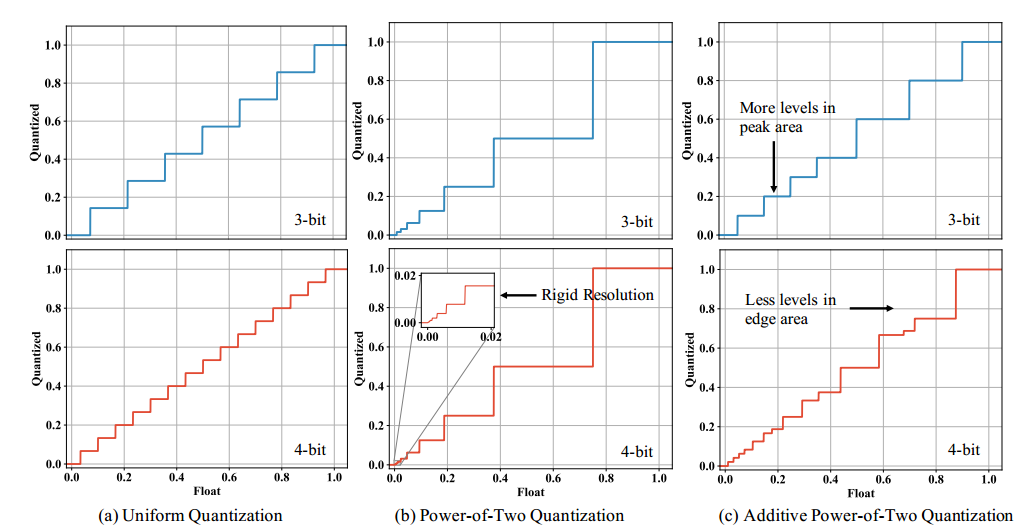因为没有mobilenet、shufflnet的实验，实验结果就不贴图了，另外因为多了一些累加，具体speedup不好说，代码开源了。

$\mathrm{pw}(r ; b, m, p)=\left\{\begin{array}{l}\operatorname{sign}(r) \times \operatorname{uni}(|r| ; b-1,0, p, 0), r \in R_{1} \\ \operatorname{sign}(r) \times \operatorname{uni}(|r| ; b-1, p, m, p), r \in R_{2}\end{array}\right.$

m是量化的值域[-m; m] (m > 0) ，$R_{1}=[-p, p]$$R_{2}=[-m,-p) \cup(p, m]$，uni表示的是均匀分布量化/线性量化。看图就能一眼看懂这个公式。